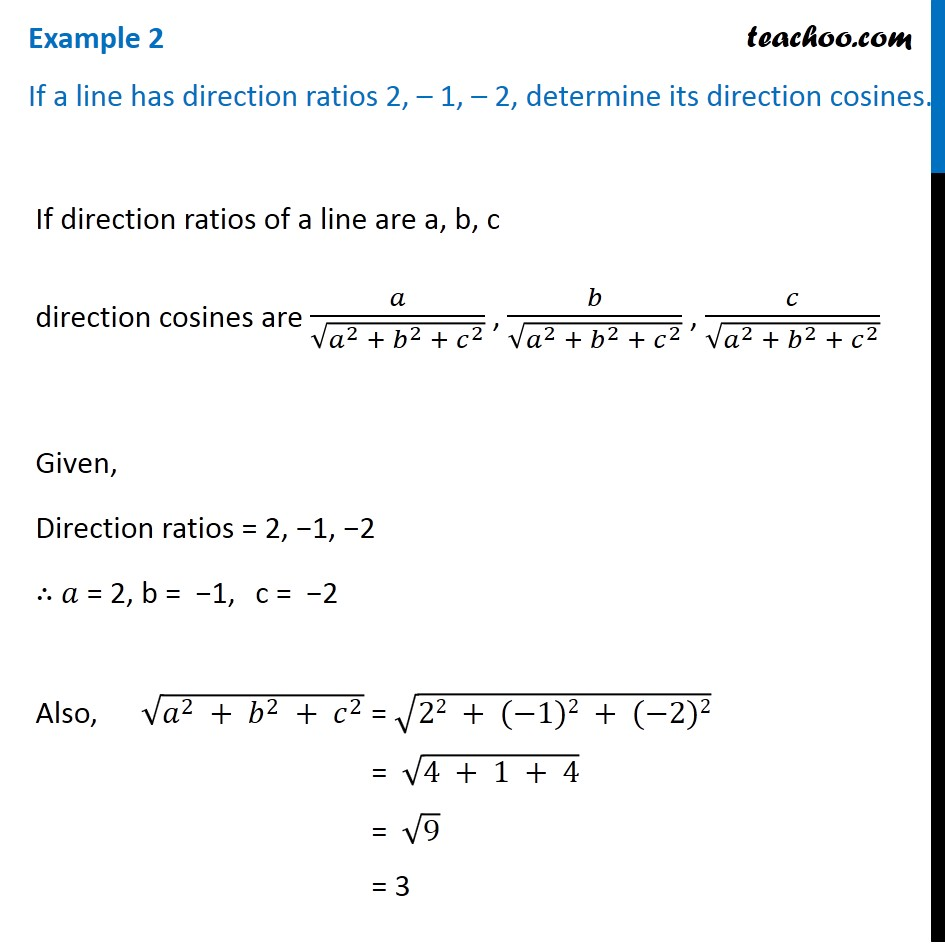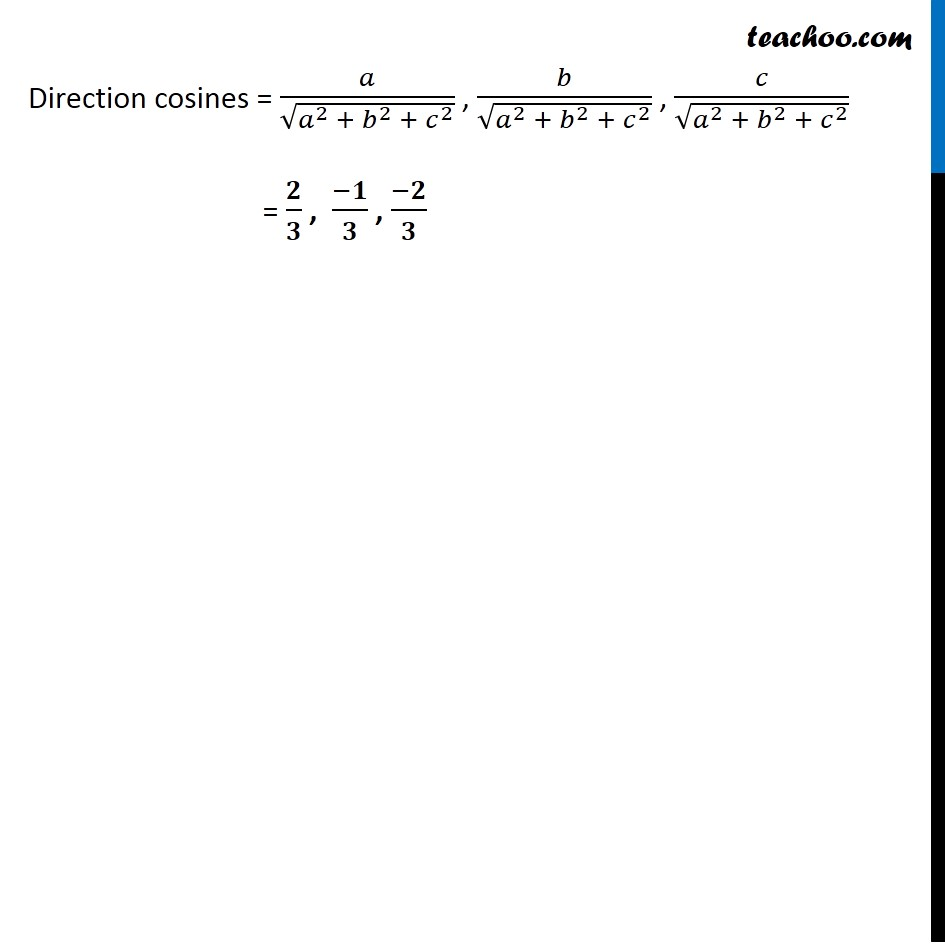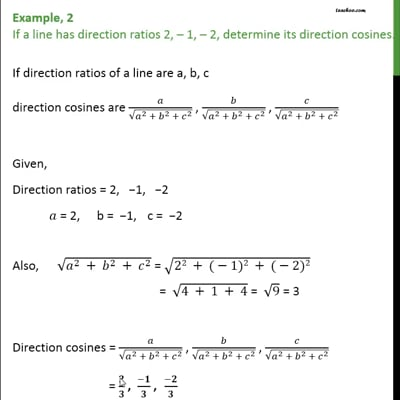Examples

Chapter 11 Class 12 Three Dimensional Geometry
Serial order wiseThis video is only available for Teachoo black users

Solve all your doubts with Teachoo Black (new monthly pack available now!)

### Transcript

Example 2 If a line has direction ratios 2, – 1, – 2, determine its direction cosines.If direction ratios of a line are a, b, c direction cosines are 𝑎/√(𝑎^2 + 𝑏^2 + 𝑐^2 ) , 𝑏/√(𝑎^2 + 𝑏^2 + 𝑐^2 ) , 𝑐/√(𝑎^2 + 𝑏^2 + 𝑐^2 ) Given, Direction ratios = 2, −1, −2 ∴ 𝑎 = 2, b = −1, c = −2 Also, √(𝑎^2 + 𝑏^2 + 𝑐^2 ) = √(22 + (−1)2 + (−2)2) = √(4 + 1 + 4) = √9 = 3 Direction cosines = 𝑎/√(𝑎^2 + 𝑏^2 + 𝑐^2 ) , 𝑏/√(𝑎^2 + 𝑏^2 + 𝑐^2 ) , 𝑐/√(𝑎^2 + 𝑏^2 + 𝑐^2 ) = 𝟐/𝟑 , (−𝟏)/𝟑 , (−𝟐)/𝟑